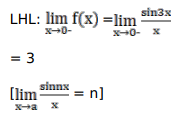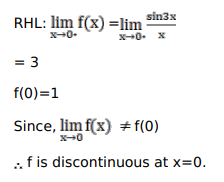# Solve this followingQuestion:

Prove that

$f(x)=\left\{\begin{array}{c}\frac{\sin 3 x}{x}, \text { when } x \neq 0 ; \\ 1, \text { when } x=0\end{array}\right.$ is discontinuous at $x=0$

Solution: